## Extract first middle and last names from full name in Excel

Supposing, you have a list of user names, now, you would like to split the full names to first, middle and last names in separated columns as following screenshot shown. This article, I will introduce some formulas for dealing with this job in Excel.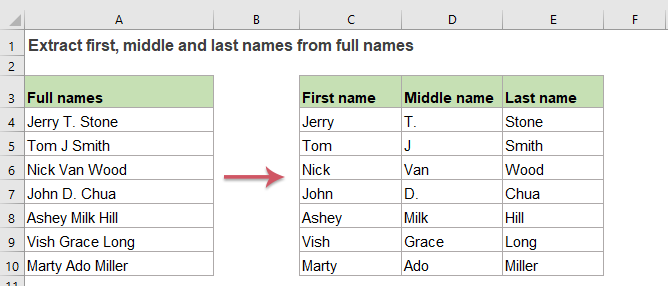#### Extract or get first names from full names in Excel

To extract or get the first names from full names, the LEFT and SEARCH function can help you, the generic syntax is:

=LEFT(name,FIND(" ",name)-1)
• name: The full name or cell reference that you want to extract first from.

Please copy or enter the following formula into a blank cell where you want to get the result:

=LEFT(A2,SEARCH(" ",A2)-1)

Then, drag the fill handle down to the cells you want to apply this formula, and all first names have been extracted as below screenshot shown: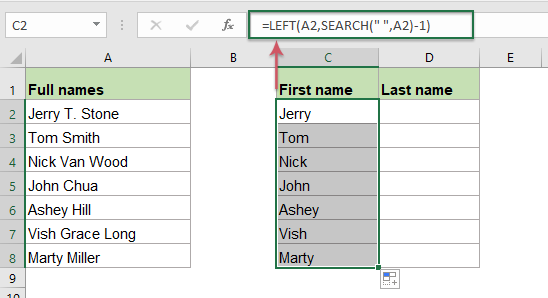##### Explanation of the formula:

SEARCH(" ",A2)-1: This SEARCH function is used to get the position of the first space character, subtract 1 means that the number of character that you want is one less than the position of the space. This will get the result: 5.

LEFT(A2,SEARCH(" ",A2)-1)= LEFT("Jerry T. Stone",5): This LEFT function will extract the text in the full name “Jerry T. Stone” from left side with 5 characters which are returned by the SEARCH function.

#### Extract or get last names from full names in Excel

To extract the last names from full names, the combination of the RIGHT, LEN, FIND, LEN and SUBSTITUTE functions can do you a favor. The generic syntax is:

=RIGHT(name,LEN(name)-FIND("*",SUBSTITUTE(name," ","*",LEN(name)-LEN(SUBSTITUTE(name," ","")))))
• name: The full name or cell reference that you want to extract last name from.

Please copy or enter the below formula into a blank cell where you want to get the last names:

=RIGHT(A2,LEN(A2)-FIND("*",SUBSTITUTE(A2," ","*",LEN(A2)-LEN(SUBSTITUTE(A2," ","")))))

And then, drag the fill handle down to the cells to fill this formula, and all the last names have been extracted from the full names, see screenshot: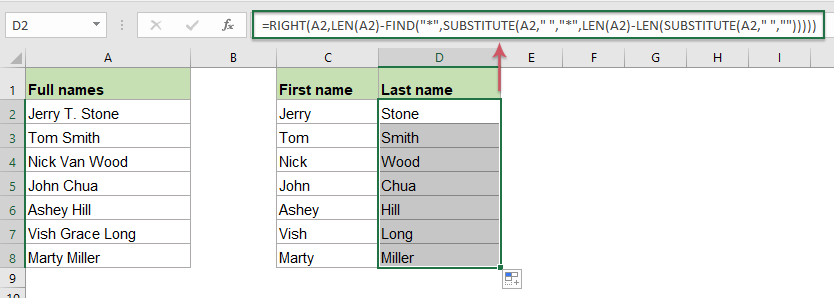##### Explanation of the formula:

1. LEN(A2)-LEN(SUBSTITUTE(A2," ","")): This part is used to get the number of the space characters in cell A2.

• LEN(A2): This LEN function returns the total number of the characters in cell A2. It will return: 14.
• SUBSTITUTE(A2," ",""): This SUBSTITUTE function is used to replace all space characters with nothing. And you will get the result as this: “JerryT.Stone”.
• LEN(SUBSTITUTE(A2," ",""): Get the total length of the full name in cell A2 without the spaces.
• LEN(A2)-LEN(SUBSTITUTE(A2,"-","")): Subtract the length of the full name without space from the total full name length to get the number of the space, and this will get 2.

2. SUBSTITUTE(A2," ","*",LEN(A2)-LEN(SUBSTITUTE(A2," ","")))= SUBSTITUTE(A2," ","*",2): This SUBSTITUTE function is used to replace the last occurrence of the space which returned by the first part formula with a * character. And you will get this result: “Jerry T.*Stone”.

3. FIND("*",SUBSTITUTE(A2," ","*",LEN(A2)-LEN(SUBSTITUTE(A2," ",""))))= FIND("*","Jerry T.*Stone" ): The FIND function will return the position of the * character in the text string which are returned by the SUBSTITUTE function, and you will get the number 9.

4. LEN(A2)-FIND("*",SUBSTITUTE(A2," ","*",LEN(A2)-LEN(SUBSTITUTE(A2," ",""))))= LEN(A2)-9: This part of the formula will get how many characters are there after the last space. This will get the number 5. This will be recognized as the num_chars argument in the RIGHT function.

5. RIGHT(A2,LEN(A2)-FIND("*",SUBSTITUTE(A2," ","*",LEN(A2)-LEN(SUBSTITUTE(A2," ","")))))=RIGHT(A2, 5): At last, the RIGHT function is used to extract 5 characters which are returned by the formula in step 4 from the right side of the text string in cell A2.

Note: If your list of full names only contains the first and last names, you can apply the following simple formula to get the last name as you need:

=RIGHT(A2,LEN(A2)-SEARCH(" ",A2,1))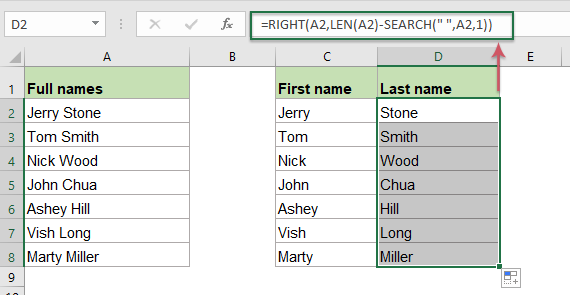#### Extract or get middle names from full names in Excel

If you need to extract the middle names from the full names, this formula which is created by the MID and SEARCH functions. The generic syntax is:

=MID(name, SEARCH(" ", name) + 1, SEARCH(" ", name, SEARCH(" ", name)+1) - SEARCH(" ", name)-1)
• name: The full name or cell reference that you want to extract middle name from.

Please enter or copy the following formula into a blank cell to output the result:

=MID(A2, SEARCH(" ", A2) + 1, SEARCH(" ", A2, SEARCH(" ", A2)+1) - SEARCH(" ", A2)-1)

Then, drag the fill handle down to cells to apply this formula, and all middle names are extracted as well, see screenshot: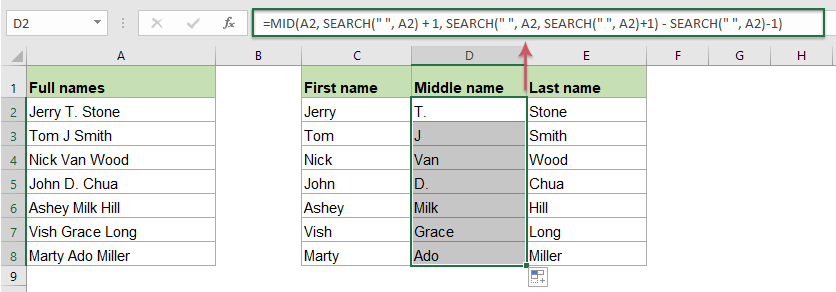##### Explanation of the formula:

To extract the middle name, first, you should find the position of both spaces between the full name, and then apply the MID function to extract the text in middle of the text string.

1. SEARCH(" ", A2) + 1: This SEARCH function will get the position of the first space, adding 1 means to start the extraction from the next character. This will get the number 7. This part is recognized as the start_num argument within the MID function.

2. SEARCH(" ", A2, SEARCH(" ", A2)+1) - SEARCH(" ", A2)-1: This part is recognized as the num_chars argument within the MID function.

• SEARCH(" ", A2, SEARCH(" ", A2)+1): This part of formula is used to get the position of the second space, it will get the number 9.
• SEARCH(" ", A2): This SEARCH function will get the position of the first space in cell A2. It will get the number 6.
• SEARCH(" ", A2, SEARCH(" ", A2)+1) - SEARCH(" ", A2)-1=9-6-1: Subtract the position of the first space from the position of the second space, and then subtract 1 from the result means to remove a leading space. And the result is 2.

3. MID(A2, SEARCH(" ", A2) + 1, SEARCH(" ", A2, SEARCH(" ", A2)+1) - SEARCH(" ", A2)-1)=MID(A2,7,2): At last, the MID function will extract 2 characters in the middle of cell A2 which starts at the seventh character.

Note: If there are only first and last names in the full names, the above formula will get an error value as below screenshot shown: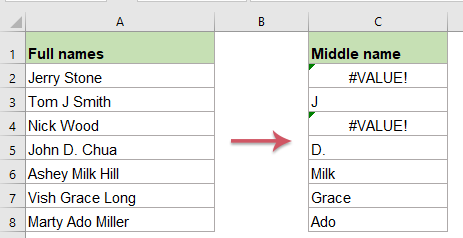To fix this problem, you should enclose the above formula into the IFERROR function, please apply the following formula:

=IFERROR(MID(A2, SEARCH(" ", A2) + 1, SEARCH(" ", A2, SEARCH(" ", A2)+1) - SEARCH(" ", A2)-1),"")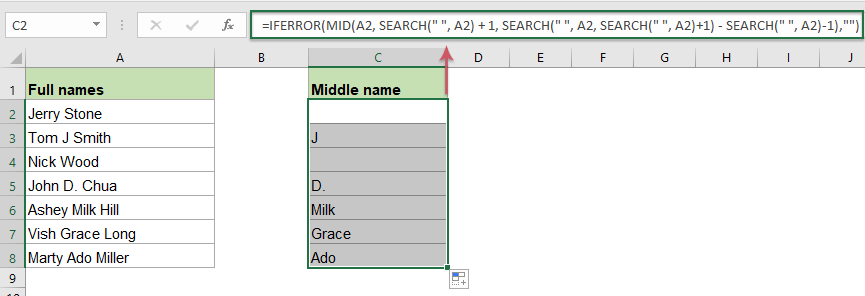#### Extract or get first, middle and last names from full names with an amazing feature

If you are painful with the above fromulas, here, I will recommend an easy tool - Kutools for Excel, with its Split Names feature, you can extract all first, midddle and last names into separate cells at once.  Click to download Kutools for Excel for free trial!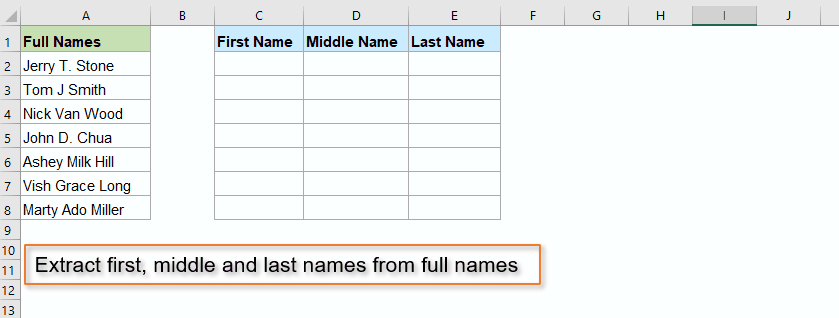#### Relative functions used:

• LEFT:
• The LEFT function extracts the given number of characters from the left side of a supplied string.
• RIGHT:
• The RIGHT function is used to extract a specific number of characters from the right side of the text string
• MID:
• The MID function is used to find and return a specific number of characters from the middle of given text string.
• LEN:
• The LEN function returns the number of characters in a text string.
• FIND:
• The FIND function is used to find a string within another string, and returns the starting position of the string inside another one.
• SEARCH:
• The SEARCH function can help you to find the position of a specific character or substring from the given text string
• SUBSTITUTE:
• The Excel SUBSTITUTE function replaces text or characters within a text string with another text or characters.

#### More articles:

• Extract first initial and last name from full name
• Supposing, you have a list of full names, now, you would like to extract the first initial and last name or first name and first character of last name from the full name as below screenshots shown. How could you deal with these tasks in Excel workbook?
• Flip Or Reverse First And Last Names In Excel List
• If you have a list of full names which are formatted as last name and first name, now, you want to flip the last and first names to first and last as below screenshot shown. This article, I will introduce some formulas for dealing with this job in Excel.
• Extract Multiple Lines From A Cell
• If you have a list of text strings which are separated by line breaks (that occurs by pressing Alt + Enter keys when entering the text), and now, you want to extract these lines of text into multiple cells as below screenshot shown. How could you solve it with a formula in Excel?
• Extract Nth Word From Text String In Excel
• If you have a list of text strings or sentences, now, you want to extract the specific nth word from the list as below screenshot shown. This article, I will introduce some methods for solving this job in Excel.

### The Best Office Productivity Tools

#### Kutools for Excel - Helps You To Stand Out From Crowd

 Popular Features: Find, Highlight or Identify Duplicates  |  Delete Blank Rows  |  Combine Columns or Cells without Losing Data  |  Round without Formula ... Super VLookup: Multiple Criteria  |  Multiple Value  |  Across Multi-Sheets  |  Fuzzy Lookup... Adv. Drop-down List: Easy Drop Down List  |  Dependent Drop Down List  |  Multi-select Drop Down List... Column Manager: Add a Specific Number of Columns  |  Move Columns  |  Toggle Visibility Status of Hidden Columns  |  Compare Columns to Select Same & Different Cells ... Featured Features: Grid Focus  |  Design View  |  Big Formula Bar  |  Workbook & Sheet Manager | Resource Library (Auto Text)  |  Date Picker  |  Combine Worksheets  |  Encrypt/Decrypt Cells  |  Send Emails by List  |  Super Filter  |  Special Filter (filter bold/italic/strikethrough...) ... Top 15 Toolsets:  12 Text Tools (Add Text, Remove Characters ...)  |  50+ Chart Types (Gantt Chart ...)  |  40+ Practical Formulas (Calculate age based on birthday ...)  |  19 Insertion Tools (Insert QR Code, Insert Picture from Path ...)  |  12 Conversion Tools (Numbers to Words, Currency Conversion ...)  |  7 Merge & Split Tools (Advanced Combine Rows, Split Excel Cells ...)  |  ... and more

Kutools for Excel Boasts Over 300 Features, Ensuring That What You Need is Just A Click Away...#### Office Tab - Enable Tabbed Reading and Editing in Microsoft Office (include Excel)

• One second to switch between dozens of open documents!
• Reduce hundreds of mouse clicks for you every day, say goodbye to mouse hand.
• Increases your productivity by 50% when viewing and editing multiple documents.
• Brings Efficient Tabs to Office (include Excel), Just Like Chrome, Edge and Firefox.No ratings yet. Be the first to rate!
This comment was minimized by the moderator on the site
عندى اسم خماسي وعاوز لغايه الاسم الرباعى فقط
This comment was minimized by the moderator on the site
Hi,